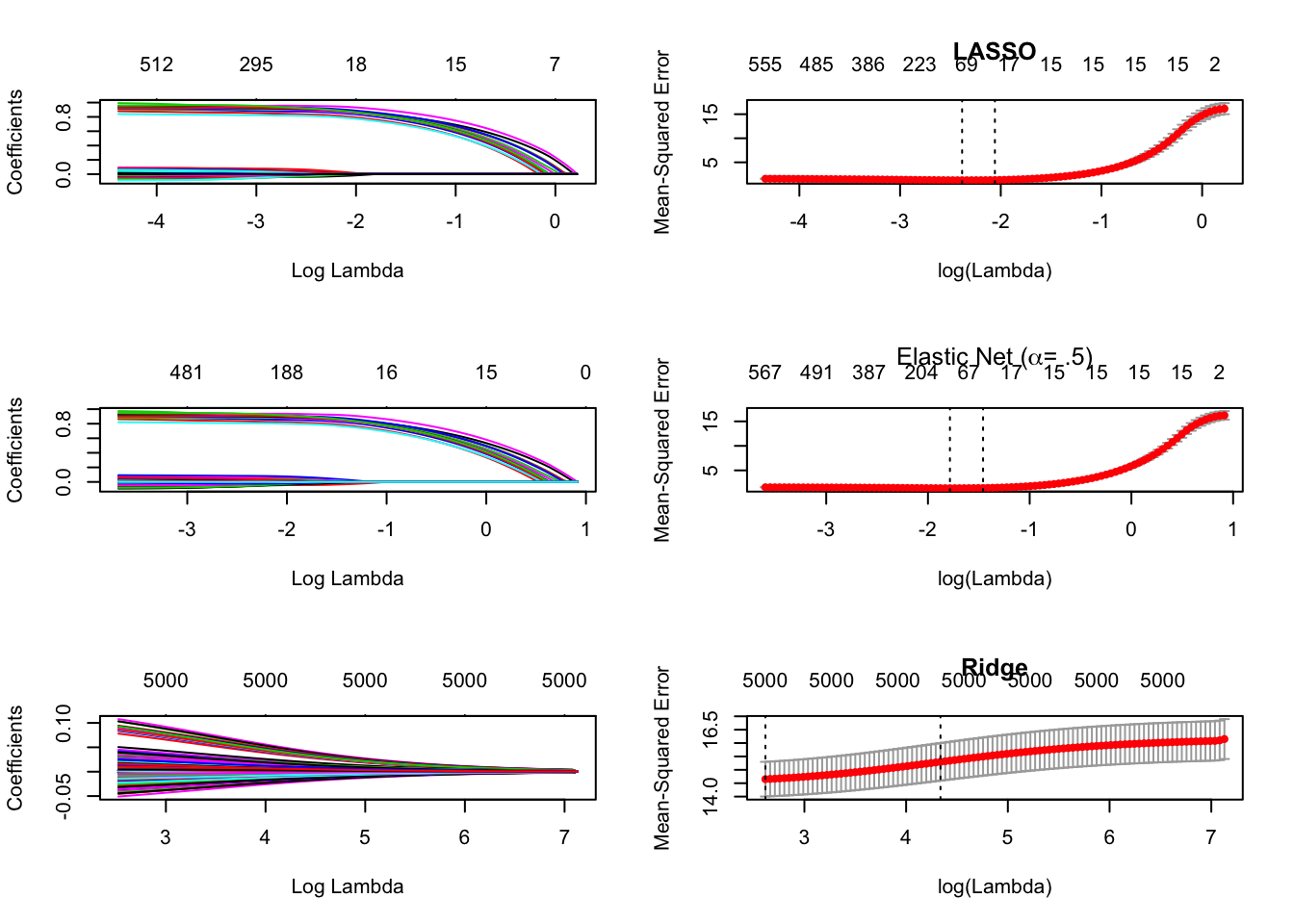The function glmnet() solves the following equation over a grid of lambda values.1

\begin{align} \min_{\beta_0,\beta} \frac{1}{N} \sum_{i=1}^{N} w_i \mathcal{L}(y_i|\beta_0+ x_i\beta) + \lambda\left[{(1-\alpha)}/{2}||\beta||_2^2 + \alpha ||\beta||_1\right] \end{align}

The elastic net penalty is represented by $$\alpha$$. When $$\alpha=1$$ the result is a lasso regression i.e. $$\lambda\left[ ||\beta||_1 \right]$$, and when $$\alpha=0$$ the result is a ridge regression i.e. $$\lambda\left[ 1/2||\beta||_2^2 \right]$$. $$\mathcal{L}(y_i|\beta_0+x_i\beta)$$ is the log-likelihood of $$y_i$$ given a linear combination of coefficients and predictors.

Since we aren’t choosing our predictors based on theoretical considerations, we need a way to determine which model is best. We can accomplish this using a variety of methods, but one of the most common is mean squared error (MSE), which is given by:

\begin{align} \frac{1}{n}\sum_{i=1}^{n} \left(\hat{Y_i} - Y_i \right)^2 \end{align}

Let’s make some fake data to test out glmnet. Create $$1000 \times 5000$$ matrix $$\mathbf{X} \sim \mathcal{N}(0,1)$$. This means we have a dataset with $$p \gg n$$, if we want to include all of the possible predictors. We need to figure out the best fitting model that includes $$1 \leq k \leq 1000$$ predictors. To make life easier in this example, create a response variable $$y$$ that is a linear combination of the first 15 variables, plus an error $$\epsilon \sim \mathcal{N}(0,1)$$ (this assumes that $$\beta \in \{ \beta_1, \ldots, \beta_{15} \} = 1$$ since we’re not multiplying our predictors by anything

set.seed(8125)
n <- 1000 # observations
p <- 5000 # predictors
x <- matrix(rnorm(n * p), nrow = n, ncol = p)
y <- apply(x[, 1:15], 1, sum) + rnorm(n) # first 15 predictors are 'true'

MSE increasingly penalizes larger deviations from the true value due to the square. Since MSE needs true and predicted values, that means we need training and test data. Randomly select 1/3 of x and y to leave out as test data.

train_rows <- sample(1:n, (2/3) * n)
x_train <- x[train_rows, ]
x_test <- x[-train_rows, ]
y_train <- y[train_rows]
y_test <- y[-train_rows]

We can easily estimate models with a variety of $$\alpha$$ values, so run glmnet() on our training data with $$\alpha = \{0,.5, 1\}$$.

library(glmnet)
fit_lasso <- glmnet(x_train, y_train, family = 'gaussian', alpha=1) # LASSO
fit_ridge <- glmnet(x_train, y_train, family = 'gaussian', alpha=0) # ridge
fit_elnet <- glmnet(x_train, y_train, family = 'gaussian', alpha=.5) # e-net

Let’s assess which of these is the best approach. And since we’re choosing models based on predictive power, let’s do so for a range of $$\alpha$$s between 0 and 1. Use the cv.glmnet() function to carry out k-fold cross validation on the training set of our fake data.

library(parallel)

# alpha values from 0 to 1
fits <- mclapply(0:10, function(x) cv.glmnet(x_train, y_train, type.measure='mse',
alpha=x/10, family='gaussian'))

The cv.glmnet() function will automatically identify the value of $$\lambda$$ that minimizes the MSE for the selected $$\alpha$$. Use plot() on the lasso, ridge, and elastic net models we ran above. Plot them next to their respective cv.glmnet() objects to see how their MSE changes with respect to different log($$\lambda$$) values.

par(mfrow=c(3,2))
plot(fit_lasso, xvar = 'lambda')
plot(fits[], main = 'LASSO')

plot(fit_elnet, xvar = 'lambda')
plot(fits[], main = expression(paste('Elastic Net (', alpha, '= .5)')))

plot(fit_ridge, xvar = 'lambda')
plot(fits[], main = 'Ridge')For the lasso and elastic net models, we can see that MSE doesn’t significantly increase until the coefficient values for our first 15 coefficients start shrinking towards 0. This tells us that we’re doing a good job of selecting relevant features without overfitting to our training data

We can extract the $$\lambda$$ value which produces a model with the lowest MSE from the cv.glmnet object, but first, let’s make sure we chose the best $$\alpha$$ value for our data. Use the predict() function and our test x and y to generate $$\hat{y}$$s for each cross validated fit_ Be sure to set s in predict() because the default is not to use the $$\lambda$$ value that produced the lowest MSE, but the entire sequence of $$\lambda$$ values tried.

# out of sample predictive accuracy
mse <- sapply(0:10, function(x) mean((predict(fits[[x + 1]], newx = x_test,
s = 'lambda.min') - y_test)^2))

# report OOS MSE for each alpha value
options(digits = 4)
kable(data.frame(alpha = (0:10)/10, mse))
alpha mse
0.0 13.983
0.1 2.385
0.2 1.600
0.3 1.388
0.4 1.292
0.5 1.247
0.6 1.215
0.7 1.199
0.8 1.188
0.9 1.175
1.0 1.165
options(digits = 2)

In this case, the model that best predicts our data is a almost a lasso with $$\alpha = 1$$. We can extract the $$lambda$$ value that produced the best fitting model from our cv.glmnet object. We can now get coefficients from the best fitting model within this model. When using coef() on the cross validated model, don’t forget to set s = 'lambda.min' since MSE is not the default. This will return a sparse matrix with the coefficient values for the coefficients included in the best fitting model (as assessed by MSE). Extract just these coefficients and their names to another object so we can see how the lasso did. str() might be helpful here…

lambda_best <- fits[]\$lambda.1se
best_coefs <- coef(fits[], s = 'lambda.min')
data.frame(name = (best_coefs@Dimnames[][best_coefs@i + 1]), coefficient = best_coefs@x)

Our lasso picked all 15 of the predictors we used to create our response variable – nice! It also picked 68 other predictors that weren’t in our model, but notice that the estimated coefficients for them (mean = 0) are much smaller than for the ‘true’ variables (mean = 0.89). This suggests that our lasso did a good job identifying important features because the implicit coefficients on the first 15 predictors are equal to 1, while the absence of the remaining predictors means their implicit coefficients are equal to 0.

If we want to assess how much explanatory power these variables are providing, we can inspect a number of plots of the model.

# fit a glmnet with our best alpha value of .9
fit_best <- glmnet(x_train, y_train, family = 'gaussian', alpha = 1)

# coefficients vs. L1 norm of coefficients
plot(fit_lasso, xvar = 'norm', label = T)